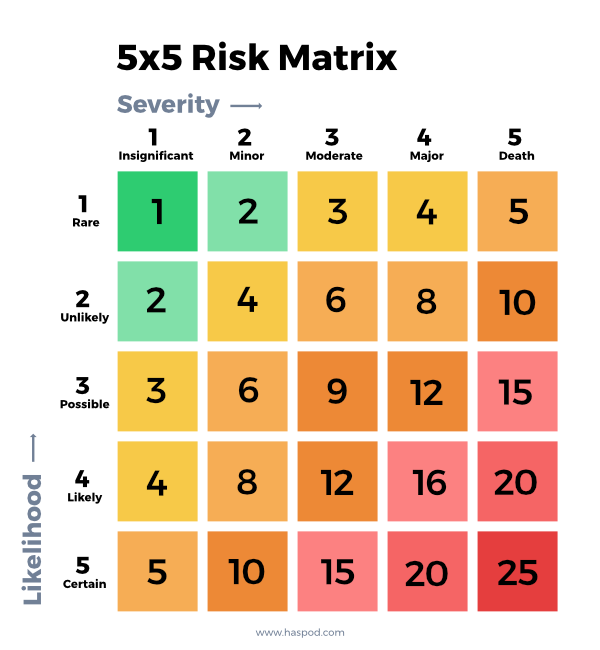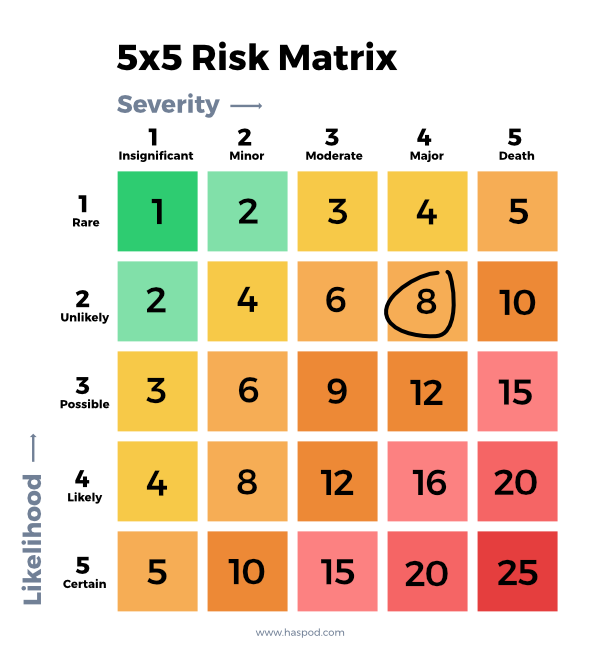2nd September, 2020

# How To Use (And Understand) A 5x5 Risk Matrix

The 5x5 risk matrix might be something you've seen in health and safety documents, in management systems or something you've heard referred to in safety briefings. But what is the 5x5 matrix? What do the numbers mean? What do the colours show? Here's how to use (and understand) a 5x5 risk matrix.Every business needs to know about risk when managing health and safety. After all, risk assessments are a legal requirement. When you're carrying out your risk assessment, at some point, you need to calculate the risk level. And since risk is a calculation of the likelihood that somebody might be harm, and how severe that harm could be, a risk matrix is a great way to measure risk.

Risk matrices typically comprise a square divided into a number of boxes, with each box representing a different underlying estimation of risk

If you're not sure how to calculate risk, don't worry, we will cover that in more detail below.

Many businesses choose to use a risk matrix for a visual representation of the risk, and because the calculation of risk is never straightforward and is always opinionated. It's not simple maths, and even if you assign numbers to risk, it's still a decision or choice which numbers to use.Even with a 5x5 risk matrix, you're using numbers. As the name suggests, your numbers will go up to 5 on each axis, giving a maximum score of 25 (5x5 = 25). But what do any of those numbers mean? Well, before we dive into assigning the numbers in the 5x5 risk matrix, you first need to know how to calculate risk. So let's cover that first.

## How To Calculate Risk

To understand the health and safety risk matrix, you first need to understand how risk is calculated. It might surprise you to know there's a little more to it than choosing if a risk is low, medium or high. Yes, there's a proper way to calculate risk, but it's fairly simple.

Risk = Likelihood x Severity

Looks pretty simple, doesn't it? The risk is how likely it is that harm will occur, against how serious that harm could be. The more likely it is that harm will happen, and the more severe the harm, the higher the risk. And before you can control risk, you need to know what level of risk you are facing.

To calculate risk, you simply need to multiply the likelihood by the severity. Well, how on earth do you do that? I'll just type "not very likely" and "broken leg" into my calculator. Nope, it's not going to work. We need another way of multiplying likelihood by severity, and we can assign numbers to each level. For example, unlikely to hurt someone could be a 1. Almost certain to cause harm could be a 5. The numbers go up on a scale for both likelihood and severity. Now we have some numbers, we can use a calculator (or your head if you're feeling especially smart today) to do some maths!

Not Very Likely (2) x Broken Leg (4) = Risk (8)

Ok, great. We have calculated some risk. But what does 8 mean? The risk level of 8 could mean anything. It needs to be put into the context of the risk scale you are using. And this is where a risk matrix comes in handy.

## Why Use A Risk Matrix

You don't have to use a 5x5 risk matrix. You don't have to use a risk matrix in your risk assessments at all. If you choose to calculate risk as Low, Medium, High for example, you can keep things simple. If the likelihood of harm occurring is low, and the severity of the harm is low, then the risk is low. But not every risk is so straight forward. What is the risk level if the likelihood is low but the severity is medium?

A risk matrix is a way of representing your risk scale in a chart (aka matrix) to show the risk level. It helps you use your scale to quickly find out if a risk is high or low.

a group of numbers or other things arranged in a rectangle that can be used to solve a problem or measure something

Instead of purely focusing on numbers, a health and safety risk matrix can use colours and a grid to show the risk level. You won't even need to use a calculator. And you could choose to do away with numbers all together if you wanted to. Like in the example below.

 Slight Moderate Extreme Low Very Low Risk Low Risk Medium Risk Medium Low Risk Medium Risk High Risk High Medium Risk High Risk Intolerable Risk

## How To Use A 5x5 Risk Matrix

Now we know how to calculate risk, and what a risk matrix is, we can apply this knowledge to the 5x5 risk matrix. Because a 5x5 risk matrix is just a way of calculating risk with 5 categories for likelihood, and 5 categories severity.

Each risk box in the matrix represents the combination of a particular level of likelihood and consequence, and can be assigned either a numerical or descriptive risk value (the risk estimate).

You could use a numeric or descriptive scale, for example:

Likelihood

1. Rare
2. Unlikely
3. Possible
4. Likely
5. Certain

Severity

1. Insignificant
2. Minor Injury
3. Moderate Injury
4. Major Injury
5. Death

Here's how the 5x5 risk matrix could look, using the above scale:Now we can calculate our risk level, from 1 (Very Low Risk) to 25 (Very High Risk) using the 5x5 risk matrix. Let's take our earlier example. A broken leg would be a major injury, but we estimated that it's not very likely to happen in the risk we are assessing. That would be unlikely (2) on our likelihood scale. And major injury (4) on our severity scale. Using the 5x5 risk matrix, we can see that gives us a medium risk.Once you know the risk level you are dealing with, you can start to think about the control measures you need. Remember, risk must be reduced as low as reasonably practicable.

If you need help calculating risk, use the free risk assessment calculator to get your risks in order.

This article was written by Emma at HASpod. Emma has over 10 years experience in health and safety and BSc (Hons) Construction Management. She is NEBOSH qualified and Tech IOSH.

## Recent posts like this...### How To Use (And Understand) A 5x5 Risk Matrix

The 5x5 risk matrix might be something you've seen in health and safety documents, in management systems or something you've heard referred to in safety briefings. But what is the 5x5 matrix? What do the numbers mean? What do the colours show? Here's how to use (and understand) a 5x5 risk matrix.### The Difference Between Site Specific And Generic Risk Assessments

If your health and safety management is being reviewed, you will often be asked for copies of site-specific risk assessments. And one of the reasons risk assessment fail the review is that they are too generic. So what do the terms site-specific risk assessments and generic risk assessments mean?### Accident Books, The Law, And How To Complete Them

Do you need an accident book at work? When should you complete it? Who fills it in? Is it a legal requirement? If you find yourself with questions about the accident book, read on, while we look to answer all of these questions and more on accident books, the law, and how to complete them.